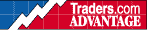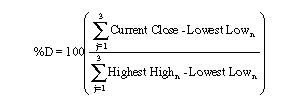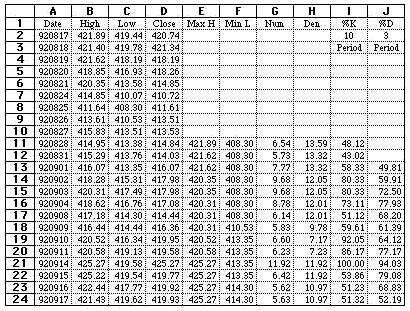HOT TOPICS LIST

INDICATORS LIST

LIST OF TOPICS

# Calculating Stochastics

06/05/01 02:40:36 PM
by Dennis D. Peterson

Security:   N/A
Position:   N/A

 The stochastics indicator calculates the relative level of today's closing price to the trading range selected by the user. Two lines are calculated, %K, which is:%K=100((Current Close-Lowest Lown) / (Highest Highn- Lowest Lown))This is the raw value of the stochastics indicator. The n period is the number of lookback days selected by the user, such as 10 days.%D is a smoothed %K. The above example smoothes the %K by calculating the ratio of the summed last three periods of the numerator of %K to the summed last three periods of the denominator of %K.In Article Figure 1, a 10-period %K and a three-period %D are presented. Column E is the highest high in the last 10 days. The formula for cell E24 is:=MAX(B15:B24)Column F is the lowest low in the last 10 days. The formula for cell for F24 is:=MIN(C15:C24)The numerator for %K is Column G. The formula for cell for G24 is:=D24 - F24Article Figure 1: Calculating stochastics using Excel. Graphic provided by: Microsoft Excel. The denominator for %K is Column H. The formula for cell for H24 is:=E24 - F24%K is calculated in column I. The formula for cell I24 is:=100*(G24/H24)Finally, %D is calculated in column J. The formula for J24 is:=100*(SUM(G22:G24)/SUM(H22:H24))The slow version of the stochastics indicator takes these steps to calculate the value of %D and then relabels %D as slow %K, then smoothes the slow %K with a three-day moving average for the %D.-- Written for Stocks & Commodities magazine by Thom HartleThis article is reprinted from Stocks & Commodities V. 10:12 (501-506) as a supplement to Dennis Peterson's April 27, 2001 article "Stochastics."

Dennis D. Peterson

Market index trading on a daily basis.

 Comments or Questions? Article Usefulness 5 (most useful) 4 3 2 1 (least useful)

Date:�10/15/01Rank:�1Comment:�I need simplicity in my life not complexity to judge whether or not to consider purchase of a stock.At age 70 I do not need all this mathmatical, algebraic etc,etc, computation to work with. I just need a few simple facts /or comments as to why a certain stock should be considerd.This is great for the college,M.B.A.,econ majors but not this lady. Thank you very much.